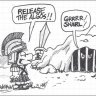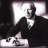# A KST indicator? ?#### markos

##### Well-known member
VIP
I beleive there is one built into TOS.

Last edited:
L

#### Likos

##### Member
2019 Donor
@markos which one and how could I format it in this matter ?

Last edited:
L

#### Likos

##### Member
2019 Donor
Can't find itLast edited:
G

#### Gildes

##### New member
VIP
@Likos

Rich (BB code):
``````# Martin Pring's KNOW SURE THING (KST) Indicator

# Daily KST Simple Moving Average

# Writen by Mike Wilgus

# fxmike.blogspot.com

# ------------------------------------------------

declare lower;

#--Input variables

input rocLength1 = 10;

input rocLength2 = 15;

input rocLength3 = 20;

input rocLength4 = 30;

def sumRocLength = rocLength1+rocLength2+rocLength3+rocLength4;

def avgLength1 = 10;

def avgLength2 = 10;

def avgLength3 = 10;

def avgLength4 = 15;

#--Calc ROC - RateOfChange(length, color norm length, price)

def ROC1 = RateOfChange(rocLength1, rocLength1, close);

def ROC2 = RateOfChange(rocLength2, rocLength2, close);

def ROC3 = RateOfChange(rocLength3, rocLength3, close);

def ROC4 = RateOfChange(rocLength4, rocLength4, close);

#--Plot lines

plot zeroLine = 0;

plot fastKST = (Average(ROC1,avgLength1)*(rocLength1/sumRocLength))+

(Average(ROC2,avgLength2)*(rocLength2/sumRocLength))+

(Average(ROC3,avgLength3)*(rocLength3/sumRocLength))+

(Average(ROC4,avgLength4)*(rocLength4/sumRocLength));

plot slowKST = Average(fastKST,rocLength1);

#--Set Colors and Style

zeroLine.SetDefaultColor(GetColor(7));

fastKST.SetDefaultColor(GetColor(5));

fastKST.SetStyle(Curve.LONG_DASH);

slowKST.SetDefaultColor(GetColor(1));

#--End Code--------------------------------------``````

Last edited by a moderator:
•markos
L

#### Likos

##### Member
2019 Donor
@Gildes This is amazing but... it's all smooshed together &#128513;Last edited:#### BenTen

Staff
VIP
@Likos Try moving it to the lower study.

Last edited:
G

2019 Donor
VIP

Last edited:
G

#### GeneHo

##### New member
2019 Donor
VIP
I mean when there is a crossover

Last edited:
N

#### Nick

##### Active member
2019 Donor
VIPHi, found a similar post from patternsmart.com on KST, i noticed that between their setting and the script posted earlier, they have included the SMA length eg sma length 5,5,5,10 in their indicator? May i know if that is necessary if so can anyone advise how to insert them into the script posted earlier?

Thank you

Last edited:#### BenTen

Staff
VIP
@Nick Not sure where those inputs came from but the Know Sure Thing indicator shared above looks correct. It could be more of a preference on the moving averages.

Last edited:#### markos

##### Well-known member
VIP
Rich (BB code):
``````#Dilbert_KnownSureThing  (AKA KST)

# V1  -  122015  - Dilbert      - 1st code cut

#13:23 dilbert: For those of you that just need 1 more moving average study where is 1 applied to the RateOfChange study.

declare lower;

input length1 = 10;

input length2 = 15;

input length3 = 20;

input length4 = 30;

input SMA1L = 10;

input SMA2L = 10;

input SMA3L = 10;

input SMA4L = 15;

input price = close;

input SignalLength = 9;

assert(length1 > 0, "'length' must be positive: " + length1);

assert(length2 > 0, "'length' must be positive: " + length2);

assert(length3 > 0, "'length' must be positive: " + length3);

assert(length4 > 0, "'length' must be positive: " + length4);

def ROC1 = if price[length1] != 0 then (price / price[length1] - 1) * 100 else 0;

def ROC2 = if price[length2] != 0 then (price / price[length2] - 1) * 100 else 0;

def ROC3 = if price[length3] != 0 then (price / price[length3] - 1) * 100 else 0;

def ROC4 = if price[length4] != 0 then (price / price[length4] - 1) * 100 else 0;

def SMA1 = SimpleMovingAvg(price,10,0);

def SMA2 = SimpleMovingAvg(price,10,0);

def SMA3 = SimpleMovingAvg(price,10,0);

def SMA4 = SimpleMovingAvg(price,15,0);

plot KST = (SMA1 * 1) + (SMA2 * 2) + (SMA3 * 3) + (SMA4 * 4);

plot SignalLine = SimpleMovingAvg(KST,SignalLength,0);

KST.SetDefaultColor(Color.Green);

SignalLine.SetDefaultColor(Color.Red);

plot BullCross = if KST crosses above SignalLine then 1 else 0;

plot BearCross = if KST crosses below SignalLine then 1 else 0;

plot Bullish = if KST > SignalLine then 1 else 0;

plot Bearish = if KST < SignalLine then 1 else 0;

BullCross.Hide();

BearCross.Hide();

Bullish.Hide();

Bearish.Hide();

# End Study``````

There are a number of KST Indicators floating around.

This just showed up in the OneNote today.

The OneNote is pinned to the top of this excellent resource, thanks to @BenTen!

Last edited by a moderator:
•J007RMC
N

#### Nick

##### Active member
2019 Donor
VIP
@markos Thank You!!

Last edited:
M

#### Miket

##### Member
is there a KST indicator crossover scan?

Last edited:#### BenTen

Staff
VIP
@GeneHo For alert, add this code to the bottom of the indicator:

Rich (BB code):
``````Alert(fastKST < slowKST and fastKST > slowKST, "Cross Above", Alert.BAR, Sound.RING);

Last edited:
M

#### Miket

##### Member
Thank you

Last edited:#### BenTen

Staff
VIP
Here is another Know Sure Thing indicator that somebody shared with me. It has different values and simple moving averages. It also has alerts as well.

Rich (BB code):
``````# ------------------------------------------------
declare lower;

#--Input variables
input rocLength1 = 17;
input rocLength2 = 12;
input rocLength3 = 20;
input rocLength4 = 25;

def sumRocLength = rocLength1+rocLength2+rocLength3+rocLength4;
input smaLength1 = 6;
input smaLength2 = 5;
input smaLength3 = 1;
input smaLength4 = 1;
input signal_length=8;
def ROC1 = RateOfChange(rocLength1, rocLength1, close);
def ROC2 = RateOfChange(rocLength2, rocLength2, close);
def ROC3 = RateOfChange(rocLength3, rocLength3, close);
def ROC4 = RateOfChange(rocLength4, rocLength4, close);

plot zeroLine = 0;

def RCMA1 =  Average(ROC1,smaLength1);
def RCMA2 =  Average(ROC2,smaLength2);
def RCMA3 =  Average(ROC3,smaLength3);
def RCMA4 =  Average(ROC4,smaLength4);

plot KST = (RCMA1* 1) + (RCMA2* 2) + (RCMA3* 3) + (RCMA4 * 4);

plot Signal_Line = Average(kst, signal_length);

KST.SetDefaultColor(Color.Lime);

Signal_Line.SetDefaultColor(Color.CYAN);

#--End Code--------------------------------------``````

Last edited:
N

#### Nick

##### Active member
2019 Donor
VIP
Hi
I am aware of the alert that we can incorporate to study, just thinking if it is possible that we can scan our watchlist and get the scan list of those stock what has is a crossover of KST and signal line?
Thanks#### markos

##### Well-known member
VIP
Hi
I am aware of the alert that we can incorporate to study, just thinking if it is possible that we can scan our watchlist and get the scan list of those stock what has is a crossover of KST and signal line?
Thanks
Sure, please go the Tutorial on How to Run the Scanner. Read through that and see the two videos.
After that, you should have no problem building it.How to read the FloatingPL indicator Questions 10